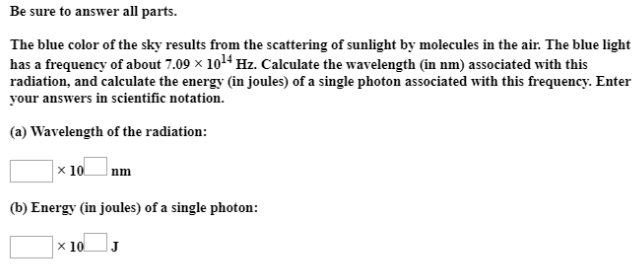# Solution: Be sure to answer all parts. The blue color of the sky results from the scattering of sunlight by molecules in the air. The blue light has a frequency of about 7.09 x 1014 Hz. Calculate the wavelength (in nm) associated with this radiation, and calculate the energy (in joules) of a single photon associated with this frequency. Enter your answers in scientific notation (a) Wavelength of the radiation: (b) Energy (in joules) of a single photon:

###### Problem

Be sure to answer all parts.

The blue color of the sky results from the scattering of sunlight by molecules in the air. The blue light has a frequency of about 7.09 x 1014 Hz. Calculate the wavelength (in nm) associated with this radiation, and calculate the energy (in joules) of a single photon associated with this frequency. Enter your answers in scientific notationView Complete Written Solution